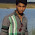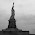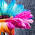## Blog Moved

This website completely moved to new domain. For latest content, visit www.programmingposts.com

## 28 Sep 2012

### C PROGRAM TO SORT MARKS OF STUDENTS WITH THEIR NAMES USING FUNCTION

/** c program to sort the marks of the students with their
names using function **/

#include<stdio.h>
#include<conio.h>
#include<string.h>
#define MAX 100 //maximum size of array
int sortArray(int); //FUNCTION DECLARATION
char Names[MAX]; //maximum
int array[MAX],new[MAX];
int main()
{
int i,size,temp1;
printf("\n>>>> PROGRAM TO SORT ARRAY WITH NAMES USING
FUNCTION <<<<\n\n");
printf("\n Enter the Number of Students: ");
scanf("%d",&size);    // reading number of students from user
if(size>100) //checks if size of array greater than 100
{
printf("\n size sholud not be more than 100 \n");
return; //terminates the program execution
}
printf("\n Enter the Names of %d Students: \n",size);
for(i=0;i<size;i++)  //loop for reading names of students
{
printf("\n Names[%d]: ",i+1);
scanf("%s",Names[i]);
}

printf("\n Enter the Marks Of Each Student: \n");

for(i=0;i<size;i++) //loop for reading marks of each student
{
printf("\n %s: ",Names[i]);
scanf("%d", &array[i]);
}
sortArray(size);  //calling function
printf("\n The Sorted elements of array are:\n");
for(i=0;i<size;i++)
{
temp1=new[i+1];
printf("\n %s : %d\n-------------",Names[i],array[i]);
}
getch();
return 0;
}

sortArray(n)     //function for sorting
{
int temp=0,i,j;   //temp var is temporary variable for sorting
char str[MAX];   //temporary string for sorting..
for(i=0;i<n;i++)
{
for(j=i+1;j<n;j++)
{

if(array[i]>array[j])
{
//swapping names and numbers for sorting
temp=array[i];        strcpy(str,Names[i]);
array[i]= array[j];   strcpy(Names[i],Names[j]);
array[j]=temp;        strcpy(Names[j],str);
}

}
}
}

Output: ( using GNU GCC Compiler with code::blocks IDE)

output 1: (here the output of the program after entering all
vlues)

output 2: (output to show if the size > 100)

1.It will be very helpful if you say how to copy the output window as a jpeg image...It will be helpful to my assignment....Anticipating a promt reply..and thanks in advance

2.@mozart : for getting image of output window take the screen shot and u can save as a image using paint software .

3.4.Good
Here is a link for C/C++ programs and pointer programs. This may be useful for you.

C/C++ Programs

5.It is nice article to improve knowledge.thank you for sharing useful info
web programming tutorial
welookups